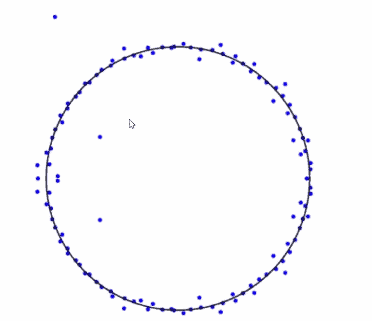# What is a Random Polynomial?

Consider a random polynomial defined by
$$f_{n}(z)=\sum_{k=0}^{n} \varepsilon_{k} z^{k}$$
where the coefficients $\varepsilon_{k}$ are IID random variables. Throughout, $\varepsilon_{k}$ will be mean-zero and finite variance. For (almost) all of this talk, they’ll be standard Gaussian random variables.

Guiding Question

How do the roots of $f_{n}$ typically behave?

### History: Real Roots

Let $N_{\mathbb{R}}\left(f_{n}\right)$ be the number of real roots, and recall $\varepsilon_{j}$ are the coefficients of the random polynomial $f_{n}$ of degree $n$.

• Waring (1782) pondered the number of real roots of a “typical cubic.”
• Bloch and Pólya (’31): When coefficients are {-1,0,1} uniformly at random, $\mathbb{E} N_{\mathbb{R}}\left(f_{n}\right)=O(\sqrt{n})$
• Littlewood and Offord (’38-48): For various different coefficients
$$\Omega(\log n / \log \log \log n)=N_{\mathbb{R}}\left(f_{n}\right)=O\left((\log n)^{2}\right)$$
• $\operatorname{Kac}(‘ 43):$ If $\varepsilon_{j} \sim \mathcal{N}(0,1),$ found exact expression for $\mathbb{E} N_{\mathbb{R}}\left(f_{n}\right)$ and
showed
$$\mathbb{E} N_{\mathbb{R}}\left(f_{n}\right) \sim \frac{2}{\pi} \log n$$

Recall $N_{\mathbb{R}}\left(f_{n}\right)$ be the number of real roots and $\varepsilon_{j}$ are the coefficients of the random polynomial $f_{n}$ of degree $n$. Kac showed:
$$\mathbb{E} N_{\mathbb{R}}\left(f_{n}\right) \sim \frac{2}{\pi} \log n$$

• $\operatorname{Kac}(‘ 48):$ Proved this for IID uniform [-1,1] .
• Erdös and Offord (’56): For {-1,1} .
• Stevens (’69); and Ibragimov and Maslova (’68-71) with the latter giving full universality (any reasonable mean zero coefficients are enough).

Edelman and Kostlan (’94): When $\varepsilon_{j} \sim N(0,1),$
$$\mathbb{E} N_{\mathbb{R}}\left(f_{n}\right)=\frac{2}{\pi} \log n+c+\frac{2}{n \pi}+O\left(1 / n^{2}\right)$$
Maslova (’74): The number of real roots has a CLT.
Dembo, Poonen, Shao and Zeitouni (’02): Found asymptotics for $\mathbb{P}\left(N_{\mathbb{R}}\left(f_{n}\right)=k\right)$ for each $k$

### History: Trigonometric Polynomials

$$X_{n}(\theta)=\sum_{k=0}^{n}\left(\varepsilon_{k} \cos (k \theta)+\varepsilon_{k}^{\prime} \sin (k \theta)\right)$$
(or similarly just sin or cos series). If $Z_{n}$ is the number of roots in $[0,2 \pi]$ and the coefficients are Gaussian then Qualls (’70) computed
$$\mathbb{E} Z_{n}=2 \sqrt{\frac{(n+1)(2 n+1)}{6}} \sim \frac{2}{\sqrt{3}} n$$
Earlier, Dunnage (’66) showed this asymptotically for just cos or sin series.
Granville and Wigman (’08) showed a CLT for $Z_{n}$ with linear variance.

### History: Complex Roots

Hammersley (’56), Šparo and Šur (’62): Most roots of a random polynomial are near the unit circle.Zeros of $f_{n}$ for $n=120$.

Ibragimov and Zaporozhets (’11): Convergence to the unit circle happens if and only if $\mathbb{E} \log \left(1+\left|\varepsilon_{1}\right|\right)<\infty$

So most roots are near the unit circle: what’s the scale?

Shepp and Vanderbei (’95) showed most roots are on the scale of $1 / n$ away from the unit circle: expected proportion within $C / n$ tends to 1 as $C \rightarrow \infty$
This is for $\varepsilon_{j} \sim \mathcal{N}(0,1) ;$ they find an exact expression for the expected number of roots in a set $A \subset \mathbb{C}$.

Peres and Virág (’05): roots uniformly bounded away from unit circle tend to a determinantal point process (for $\left.\varepsilon_{j} \sim \mathcal{N}_{\mathbb{C}}(0,1)\right)$

Left is a determinantal point process, right is a Poisson process (taken from Peres and Virág). As $n \rightarrow \infty$ (or $n=\infty),$ density of having zeros at
$z_{1}, \ldots, z_{k}$ converges to
$$\pi^{-k} \operatorname{det}\left(\frac{1}{\left(1-z_{i} \bar{z}{j}\right)^{2}}\right){i, j}$$

### This is (sorta obviously) not universal

Peres and Virág implies that when $\varepsilon_{j} \sim \mathcal{N}_{\mathbb{C}}(0,1)$ roots can be arbitrarily close to 0 and $\infty$

(Complex gaussian coefficients, degree $=120$ (and lots of sampling))
Conversely, if $\varepsilon_{j} \in{-1,1}$ then all roots have $|z| \in[1 / 2,2]$ so behavior far from unit circle is not universal.

### Classic explanation why roots converge to the unit circle

Here’s a quick and dirty sketch (that’s pretty close to a proof): Complex analysis (the argument principle) says the number of roots $\zeta$ with $|\zeta| \leq r$
$$\frac{1}{2 \pi i} \oint_{|z|=r} \frac{f_{n}^{\prime}(z)}{f_{n}(z)} d z$$
For $|z|>1,$ since the coefficients of $f_{n}$ are random, we have
$$\begin{array}{l} f_{n}^{\prime}(z)=n \varepsilon_{n} z^{n-1}+\cdots \varepsilon_{1} \approx n \varepsilon_{n} z^{n-1} \ f_{n}(z)=\varepsilon_{n} z^{n}+\cdots+\varepsilon_{0} \approx \varepsilon_{n} z^{n} \end{array}$$
So the number of roots $\zeta$ with $|\zeta| \leq r$ for $r>1$ is like
$$\frac{1}{2 \pi i} \oint_{|z|=r} \frac{f_{n}^{\prime}(z)}{f_{n}(z)} d z \approx \frac{1}{2 \pi i} \oint_{|z|=r} \frac{n}{z} d z=n$$
Symmetrically, if $r<1$ then the number of roots with $|\zeta| \leq r$ is $\approx 0$ (To turn this into a proof, integrate $\Delta \log \left|f_{n}(z)\right|$ to get the number of roots instead of $\frac{f_{n}^{\prime}}{f_{n}}$.)

### A different heuristic: Overview of the Proof

• A complex zero $z$ is a point where $\operatorname{Re}\left(f_{n}(z)\right)=0$ and $\operatorname{Im}\left(f_{n}(z)\right)=0$ simultaneously.
• Let $X_{r}(\theta)=\operatorname{Re}\left(f_{n}\left(r e^{i \theta}\right)\right)$. Then
$$X_{1}(\theta)=\sum_{k=0}^{n} \varepsilon_{k} \cos (k \theta)$$
typically has $\sim \frac{2}{\sqrt{3}} n$ zeros (we’ll discuss this more in a moment). The same is true for $Y_{r}(\theta)=\operatorname{Im}\left(f_{n}\left(r e^{i \theta}\right)\right)$.
• With this in mind, think of placing $\approx n$ red balls uniformly at random on the unit circle and $\approx n$ blue balls similarly (representing the roots of real and imaginary parts respectively).
• A typical ball is within $O(1 / n)$ of a ball of the other color, but a few will be within $O\left(1 / n^{2}\right)$ of the other color.
• If we look at the circle of radius $1+h / n^{2}$ for some $h \in \mathbb{R},$ then each ball moves roughly $O\left(h / n^{2}\right)$ implying some red and blue balls will collide (i.e. a zero occurs).
• Since most balls are within $O(1 / n)$ of a different colored ball, this also suggests most pairs collide within time $O(1 / n)$.